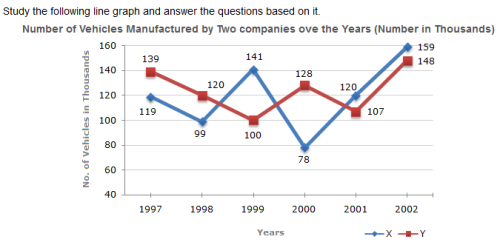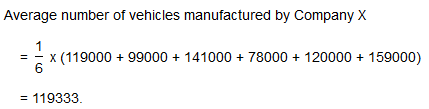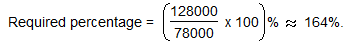Courses

# Test: Line Charts- 3

## 5 Questions MCQ Test UPSC CSAT Preparation | Test: Line Charts- 3

Description
This mock test of Test: Line Charts- 3 for Banking Exams helps you for every Banking Exams entrance exam. This contains 5 Multiple Choice Questions for Banking Exams Test: Line Charts- 3 (mcq) to study with solutions a complete question bank. The solved questions answers in this Test: Line Charts- 3 quiz give you a good mix of easy questions and tough questions. Banking Exams students definitely take this Test: Line Charts- 3 exercise for a better result in the exam. You can find other Test: Line Charts- 3 extra questions, long questions & short questions for Banking Exams on EduRev as well by searching above.
QUESTION: 1

###What is the difference between the number of vehicles manufactured by Company Y in 2000 and 2001 ?

Solution:

Required difference = (128000 - 107000) = 21000.

QUESTION: 2

###What is the difference between the total productions of the two Companies in the given years ?

Solution:

From the line-graph it is clear that the productions of Company X in the years 1997, 1998, 1999, 2000, 2001 and 2002 are 119000, 99000, 141000, 78000, 120000 and 159000 and those of Company Y are 139000, 120000,100000, 128000, 107000 and 148000 respectively.

Total production of Company X from 1997 to 2002

= 119000 + 99000 + 141000 + 78000 + 120000 + 159000

= 716000.

and total production of Company Y from 1997 to 2002

= 139000 + 120000 + 100000 + 128000 + 107000 + 148000

= 742000.

Difference = (742000 - 716000) = 26000.

QUESTION: 3

###What is the average numbers of vehicles manufactured by Company X over the given period ? (rounded off to nearest integer)

Solution:QUESTION: 4In which of the following years, the difference between the productions of Companies X and Y was the maximum among the given years ?

Solution:

The difference between the productions of Companies X and Y in various years are:

For 1997 (139000 - 119000) = 20000.

For 1998 (120000 - 99000) = 21000.

For 1999 (141000 - 100000) = 41000.

For 2000 (128000 - 78000) = 50000.

For 2001 (120000 - 107000) = 13000.

For 2002 (159000 - 148000) = 11000.

Clearly, maximum difference was in 2000.

QUESTION: 5The production of Company Y in 2000 was approximately what percent of the production of Company X in the same year ?

Solution: